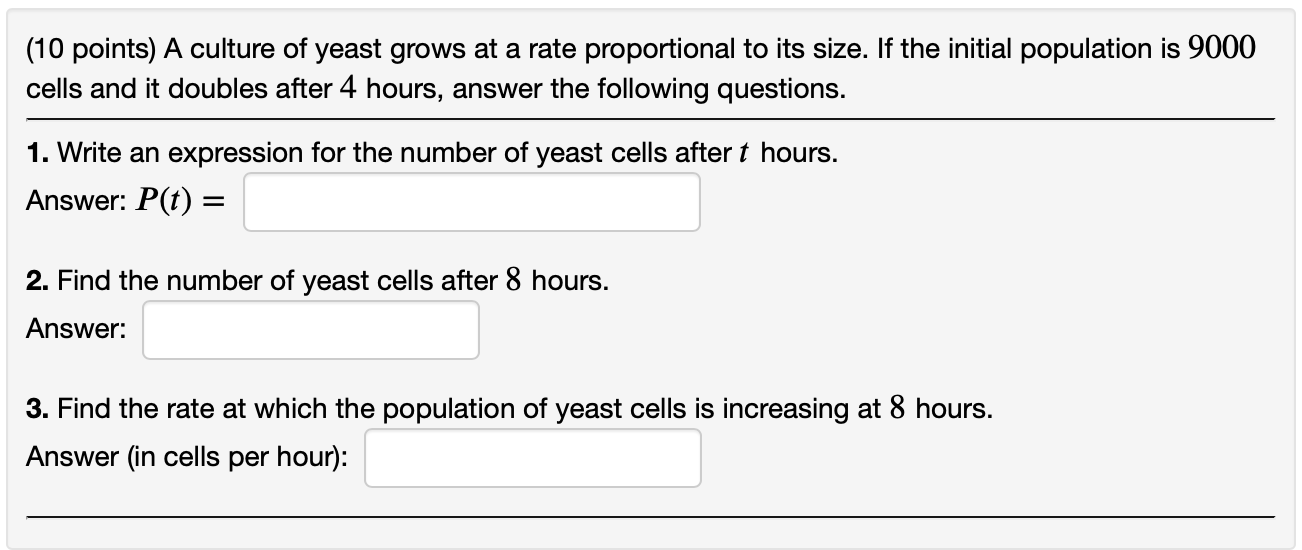Home / Answered Questions / Other / 10-points-a-culture-of-yeast-grows-at-a-rate-proportional-to-its-size-if-the-initial-population-is-9-aw614

# (Solved): (10 Points) A Culture Of Yeast Grows At A Rate Proportional To Its Size. If The Initial Population I...(10 points) A culture of yeast grows at a rate proportional to its size. If the initial population is 9000 cells and it doubles after 4 hours, answer the following questions. 1. Write an expression for the number of yeast cells after t hours. Answer: P(t) = 2. Find the number of yeast cells after 8 hours. Answer: 3. Find the rate at which the population of yeast cells is increasing at 8 hours. Answer (in cells per hour):

We have an Answer from Expert